Ex 13.2

Chapter 13 Class 9 Surface Areas and Volumes
Serial order wise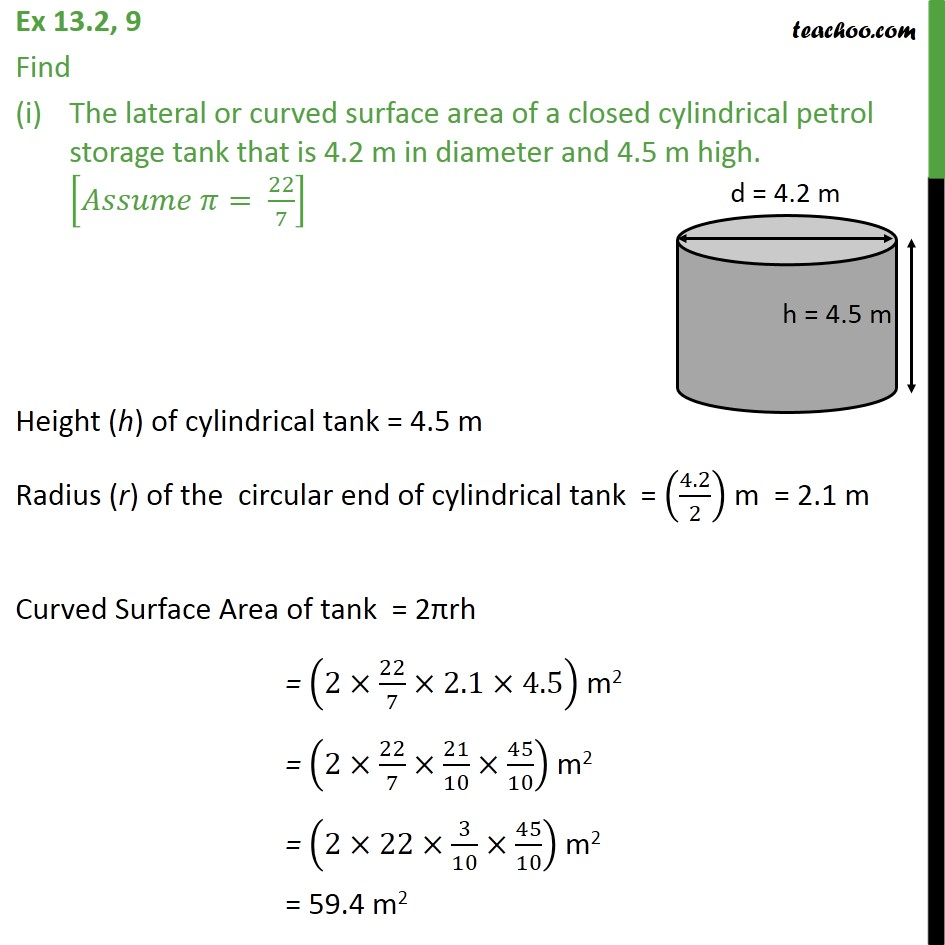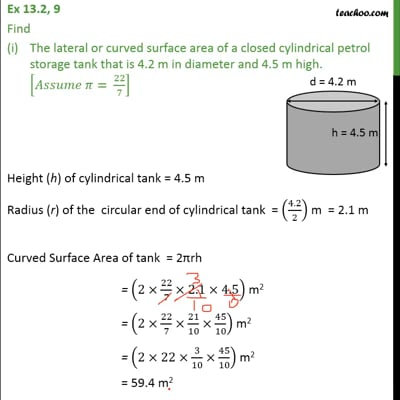This video is only available for Teachoo black users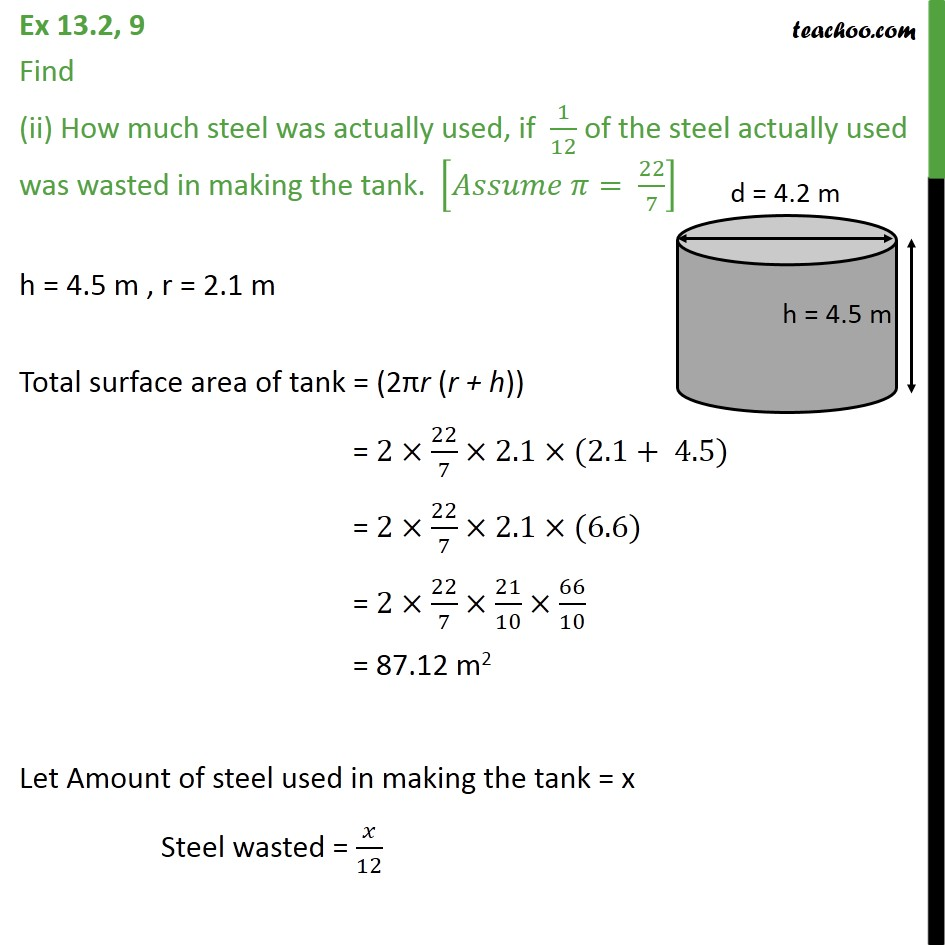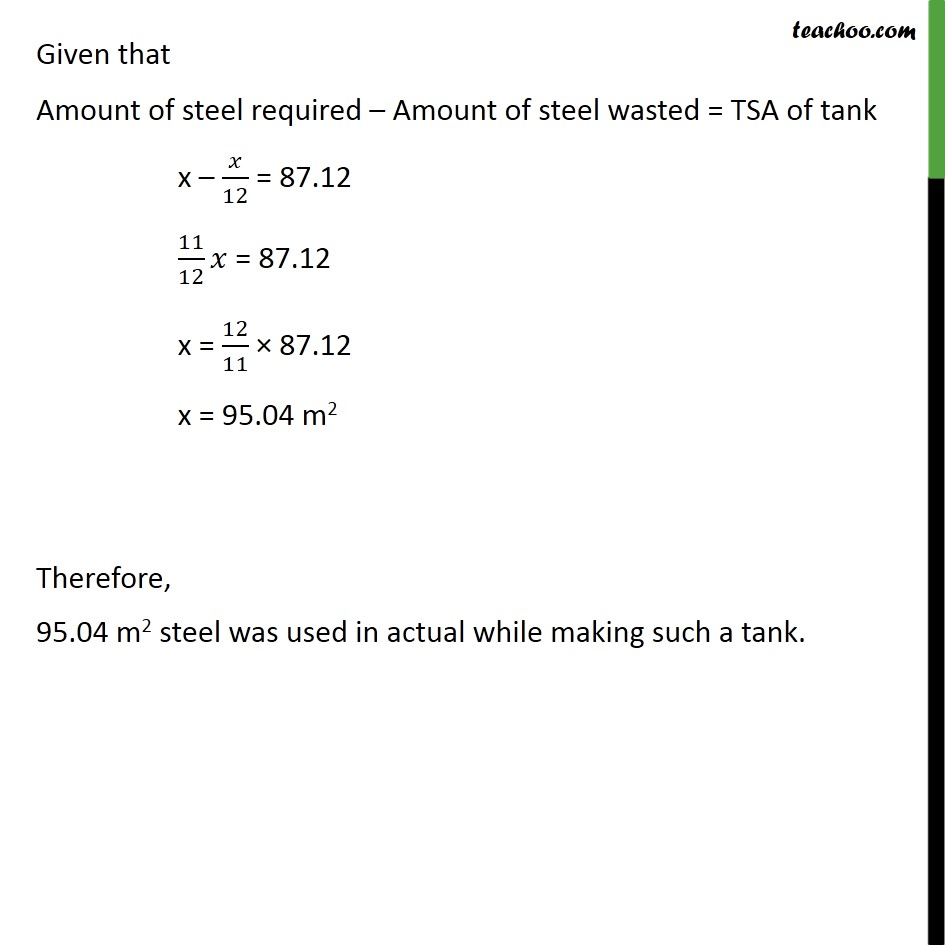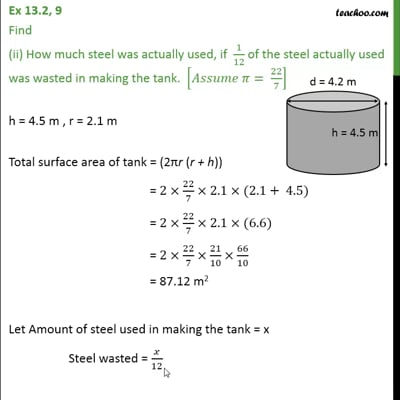This video is only available for Teachoo black users

Maths Crash Course - Live lectures + all videos + Real time Doubt solving!

### Transcript

Ex 13.2, 9 Find The lateral or curved surface area of a closed cylindrical petrol storage tank that is 4.2 m in diameter and 4.5 m high. [𝐴𝑠𝑠𝑢𝑚𝑒 𝜋= 22/7] Height (h) of cylindrical tank = 4.5 m Radius (r) of the circular end of cylindrical tank = (4.2/2) m = 2.1 m Curved Surface Area of tank = 2πrh = (2×22/7×2.1×4.5) m2 = (2×22/7×21/10×45/10) m2 = (2×22×3/10×45/10) m2 = 59.4 m2 Ex 13.2, 9 Find (ii) How much steel was actually used, if 1/12 of the steel actually used was wasted in making the tank. [𝐴𝑠𝑠𝑢𝑚𝑒 𝜋= 22/7] h = 4.5 m , r = 2.1 m Total surface area of tank = (2πr (r + h)) = 2×22/7×2.1×(2.1+ 4.5) = 2×22/7×2.1×(6.6) = 2×22/7×21/10×66/10 = 87.12 m2 Let Amount of steel used in making the tank = x Steel wasted = 𝑥/12 Given that Amount of steel required – Amount of steel wasted = TSA of tank x – 𝑥/12 = 87.12 11/12 𝑥 = 87.12 x = 12/11 × 87.12 x = 95.04 m2 Therefore, 95.04 m2 steel was used in actual while making such a tank.